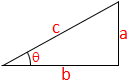# Arccos Calculator

In mathematics, the inverse trigonometric functions are the inverse functions of the trigonometric functions. Specifically, the arccos is the inverse of the cosine. It is normally represented by arccos(θ) or cos-1(θ).arccos = ?